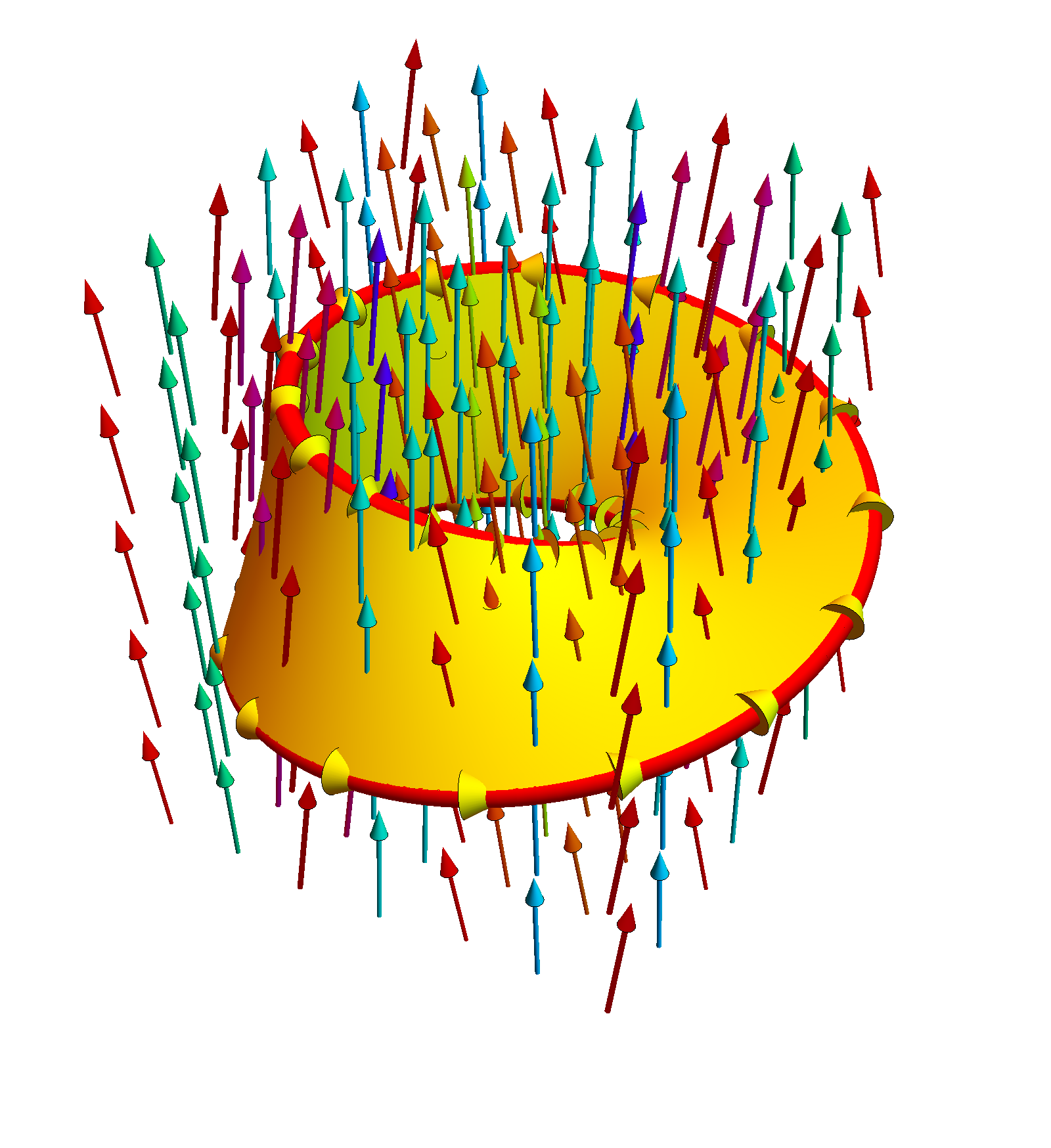# Flux through Moebius Strip

The Moebius strip is a surface which has no orientation. This can be a bit puzzling, because we can well write down a parametrization r = [(2 + v cos(u/2)) cos(u), (2 + v cos(u/2)) sin(u), v sin(u/2)], where u goes from 0 to 2π and v goes from -1 to 1. If one looks at the animation, where the bound for u is changed from 0 to &2pi; one can see what happens: the orientations are off at the end, when the two ends merge.
```r = {(2 + v Cos[u/2]) Cos[u], (2 + v Cos[u/2]) Sin[u], v Sin[u/2]};
n = Cross[D[r, u], D[r, v]];
Integrate[Sqrt[n.n], {u, 0, 2 Pi}, {v, -1, 1}]
```
 Direct Media Links: Webm, Ogg Ipod ; We can compute the surface area of the strip (the above computation shows its value is about 25.4131... We also compute the flux of the curl of a vector field through the surface and hope to apply Stokes theorem. Now this is a bit puzzling, as the normal vector flips if we parametrize from 0 to 2π. If we parametrize with 0 < u < 4π, then we cover both sides of the strip and get zero. But if we integrate from 0 to 2π then the result is twice the line integral along the cut and boundary curve as if we cut the strip, we get a surface which is orientable and for which Stokes theorem applies.